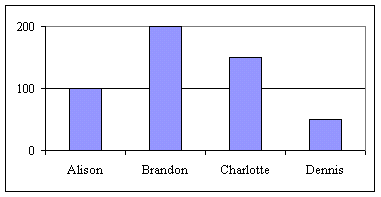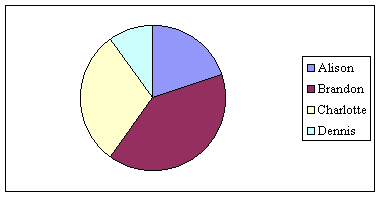# GMAT Problem Solving Questions

Below are a sample set of problem solving questions similar to those found in the quantitative section of the GMAT. Due to the relatively small number of questions published here, we strongly recommend that you only use these to augment your GMAT study preparations.

1) If you roll a fair-sided dice twice, what is the probability the dice will land on the same number both time?

1. 1/3
2. 1/6
3. 1/12
4. 1/24
5. 1/36

2) The end of a blade on an airplane propeller is 10 feet from the center. If the propeller spins at the rate of 1,320 revolutions per second, how many miles will the tip of the blade travel in one minute? (1 mile = 5,280 feet)

1. 200p
2. 240p
3. 300p
4. 480p
5. 1,200p

Note to Netscape browser users: The answer choices are 200 “pi” through 1,200 “pi”. (The “pi” symbol inexplicably does not show up in certain Netscape browsers.)3) The two graphs above depict the number of units sold by ABC Company’s four salespeople. How many degrees is the angle for the sector of the pie chart representing Alison?

1. 20
2. 36
3. 60
4. 72
5. 108

4) If a* = (2a + 6)/4, and a = 3b + 1, then a* equals

1. 0.5b + 1.5
2. 1.5b + 2
3. 1.5b – 2
4. 6b + 7
5. b – 1/3

5) Two years ago, Tim was three times as old as Susie. Today he is twice as old as Susie. How old is Tim today?

1. 2
2. 4
3. 6
4. 8
5. Can not be determined based on the information given.

6) Which of the following numbers is evenly divisible by 2, 3, 4, and 5, but not by 9?

1. 15,215
2. 21,896
3. 47,630
4. 60,840
5. 78,420